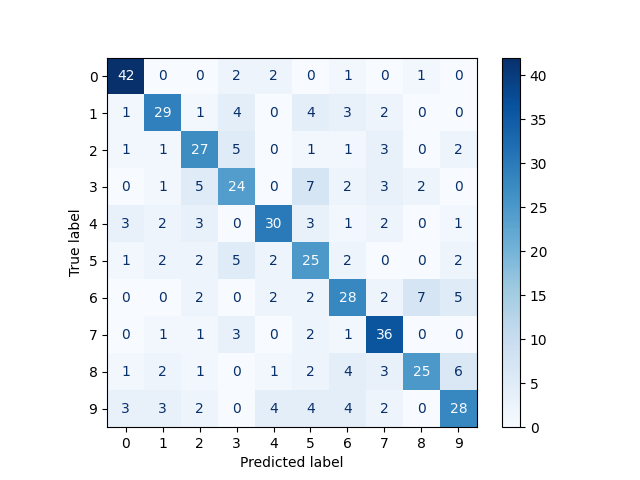# Fisher vector feature encoding#

A Fisher vector is an image feature encoding and quantization technique that can be seen as a soft or probabilistic version of the popular bag-of-visual-words or VLAD algorithms. Images are modelled using a visual vocabulary which is estimated using a K-mode Gaussian mixture model trained on low-level image features such as SIFT or ORB descriptors. The Fisher vector itself is a concatenation of the gradients of the Gaussian mixture model (GMM) with respect to its parameters - mixture weights, means, and covariance matrices.

In this example, we compute Fisher vectors for the digits dataset in scikit-learn, and train a classifier on these representations.

Please note that scikit-learn is required to run this example.```              precision    recall  f1-score   support

0       0.87      0.91      0.89        45
1       0.63      0.79      0.70        48
2       0.64      0.71      0.67        45
3       0.64      0.68      0.66        34
4       0.69      0.62      0.66        40
5       0.61      0.62      0.62        48
6       0.58      0.52      0.55        48
7       0.63      0.69      0.66        42
8       0.73      0.61      0.67        49
9       0.52      0.43      0.47        51

accuracy                           0.66       450
macro avg       0.66      0.66      0.66       450
weighted avg       0.65      0.66      0.65       450
```

```from matplotlib import pyplot as plt
import numpy as np
from sklearn.metrics import classification_report, ConfusionMatrixDisplay
from sklearn.model_selection import train_test_split
from sklearn.svm import LinearSVC

from skimage.transform import resize
from skimage.feature import fisher_vector, ORB, learn_gmm

images = data.images
targets = data.target

# Resize images so that ORB detects interest points for all images
images = np.array([resize(image, (80, 80)) for image in images])

# Compute ORB descriptors for each image
descriptors = []
for image in images:
detector_extractor = ORB(n_keypoints=5, harris_k=0.01)
detector_extractor.detect_and_extract(image)
descriptors.append(detector_extractor.descriptors.astype('float32'))

# Split the data into training and testing subsets
train_descriptors, test_descriptors, train_targets, test_targets = \
train_test_split(descriptors, targets)

# Train a K-mode GMM
k = 16
gmm = learn_gmm(train_descriptors, n_modes=k)

# Compute the Fisher vectors
training_fvs = np.array([
fisher_vector(descriptor_mat, gmm)
for descriptor_mat in train_descriptors
])

testing_fvs = np.array([
fisher_vector(descriptor_mat, gmm)
for descriptor_mat in test_descriptors
])

svm = LinearSVC().fit(training_fvs, train_targets)

predictions = svm.predict(testing_fvs)

print(classification_report(test_targets, predictions))

ConfusionMatrixDisplay.from_estimator(
svm,
testing_fvs,
test_targets,
cmap=plt.cm.Blues,
)

plt.show()
```

Total running time of the script: ( 0 minutes 57.377 seconds)

Gallery generated by Sphinx-Gallery